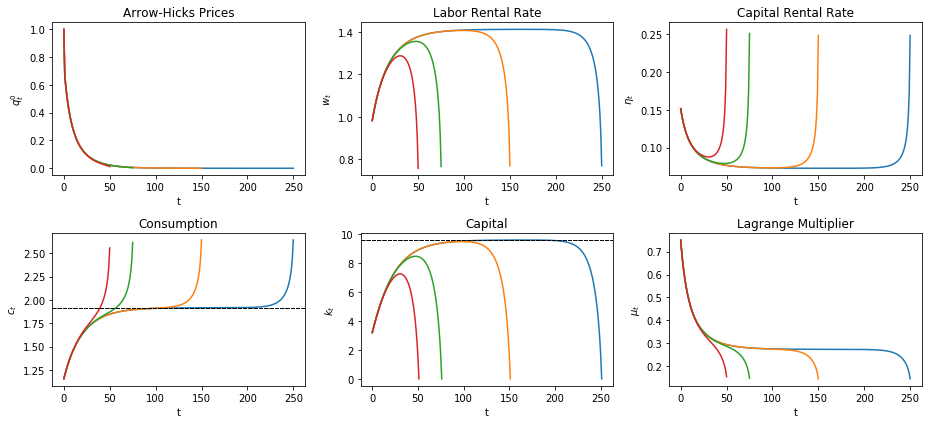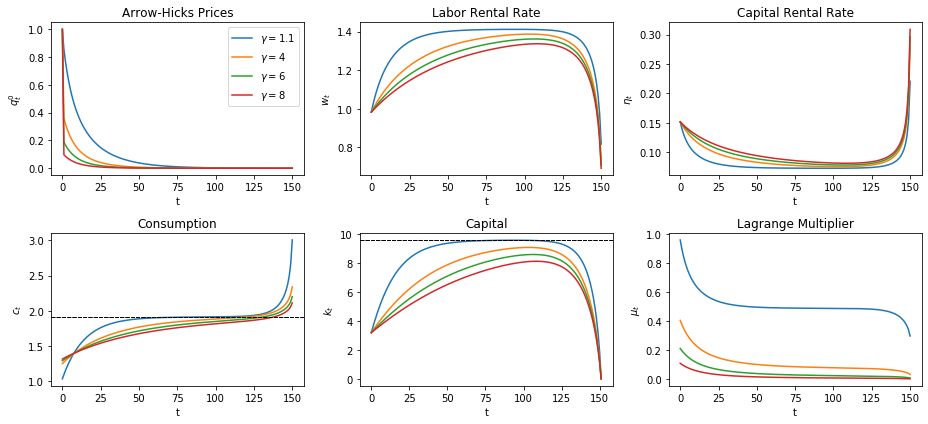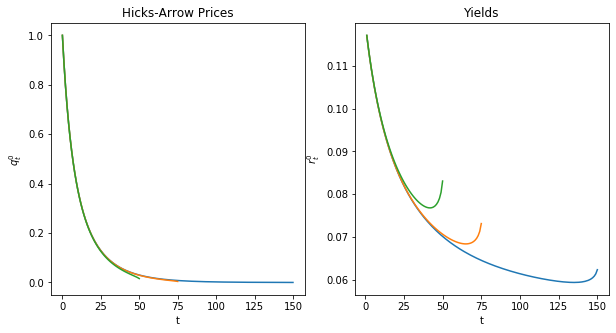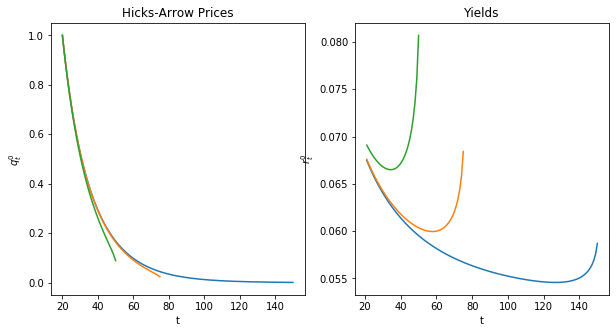# Cass-Koopmans Competitive Equilibrium¶

## Overview¶

This lecture continues our analysis in this lecture Cass-Koopmans Planning Model about the model that Tjalling Koopmans [Koo65] and David Cass [Cas65] used to study optimal growth.

This lecture illustrates what is, in fact, a more general connection between a planned economy and an economy organized as a competitive equilibrium.

The earlier lecture Cass-Koopmans Planning Model studied a planning problem and used ideas including

• A min-max problem for solving the planning problem.
• A shooting algorithm for solving difference equations subject to initial and terminal conditions.
• A turnpike property that describes optimal paths for long-but-finite horizon economies.

The present lecture uses additional ideas including

• Hicks-Arrow prices named after John R. Hicks and Kenneth Arrow.
• A connection between some Lagrange multipliers in the min-max problem and the Hicks-Arrow prices.
• A Big $K$ , little $k$ trick widely used in macroeconomic dynamics.
• A non-stochastic version of a theory of the term structure of interest rates.
• An intimate connection between the cases for the optimality of two competing visions of good ways to organize an economy, namely:
• socialism in which a central planner commands the allocation of resources, and
• capitalism (also known as a market economy) in which competitive equilibrium prices induce individual consumers and producers to choose a socially optimal allocation as an unintended consequence of their selfish decisions

Let’s start with some standard imports:

In :
from numba import njit, float64
from numba.experimental import jitclass
import numpy as np
import matplotlib.pyplot as plt
%matplotlib inline


## Review of Cass-Koopmans Model¶

The physical setting is identical with that in Cass-Koopmans Planning Model.

Time is discrete and takes values $t = 0, 1 , \ldots, T$.

A single good can either be consumed or invested in physical capital.

The consumption good is not durable and depreciates completely if not consumed immediately.

The capital good is durable but partially depreciates each period at a constant rate.

We let $C_t$ be a nondurable consumption good at time t.

Let $K_t$ be the stock of physical capital at time t.

Let $\vec{C}$ = $\{C_0,\dots, C_T\}$ and $\vec{K}$ = $\{K_0,\dots,K_{T+1}\}$.

A representative household is endowed with one unit of labor at each $t$ and likes the consumption good at each $t$.

The representative household inelastically supplies a single unit of labor $N_t$ at each $t$, so that $N_t =1 \text{ for all } t \in [0,T]$.

The representative household has preferences over consumption bundles ordered by the utility functional:

$$U(\vec{C}) = \sum_{t=0}^{T} \beta^t \frac{C_t^{1-\gamma}}{1-\gamma}$$

where $\beta \in (0,1)$ is a discount factor and $\gamma >0$ governs the curvature of the one-period utility function.

We assume that $K_0 > 0$.

There is an economy-wide production function

$$F(K_t,N_t) = A K_t^{\alpha}N_t^{1-\alpha}$$

with $0 < \alpha<1$, $A > 0$.

A feasible allocation $\vec{C}, \vec{K}$ satisfies

$$C_t + K_{t+1} \leq F(K_t,N_t) + (1-\delta) K_t, \quad \text{for all } t \in [0, T]$$

where $\delta \in (0,1)$ is a depreciation rate of capital.

### Planning Problem¶

In this lecture Cass-Koopmans Planning Model, we studied a problem in which a planner chooses an allocation $\{\vec{C},\vec{K}\}$ to maximize (1) subject to (4).

The allocation that solves the planning problem plays an important role in a competitive equilibrium as we shall see below.

## Competitive Equilibrium¶

We now study a decentralized version of the economy.

It shares the same technology and preference structure as the planned economy studied in this lecture Cass-Koopmans Planning Model.

But now there is no planner.

Market prices adjust to reconcile distinct decisions that are made separately by a representative household and a representative firm.

There is a representative consumer who has the same preferences over consumption plans as did the consumer in the planned economy.

Instead of being told what to consume and save by a planner, the household chooses for itself subject to a budget constraint

• At each time $t$, the household receives wages and rentals of capital from a firm – these comprise its income at time $t$.
• The consumer decides how much income to allocate to consumption or to savings.
• The household can save either by acquiring additional physical capital (it trades one for one with time $t$ consumption) or by acquiring claims on consumption at dates other than $t$.
• The household owns all physical capital and labor and rents them to the firm.
• The household consumes, supplies labor, and invests in physical capital.
• A profit-maximizing representative firm operates the production technology.
• The firm rents labor and capital each period from the representative household and sells its output each period to the household.
• The representative household and the representative firm are both price takers who believe that prices are not affected by their choices

Note: We can think of there being a large number $M$ of identical representative consumers and $M$ identical representative firms.

## Market Structure¶

The representative household and the representative firm are both price takers.

The household owns both factors of production, namely, labor and physical capital.

Each period, the firm rents both factors from the household.

There is a single grand competitive market in which a household can trade date $0$ goods for goods at all other dates $t=1, 2, \ldots, T$.

### Prices¶

There are sequences of prices $\{w_t,\eta_t\}_{t=0}^T= \{\vec{w}, \vec{\eta} \}$ where $w_t$ is a wage or rental rate for labor at time $t$ and $\eta_t$ is a rental rate for capital at time $t$.

In addition there is are intertemporal prices that work as follows.

Let $q^0_t$ be the price of a good at date $t$ relative to a good at date $0$.

We call $\{q^0_t\}_{t=0}^T$ a vector of Hicks-Arrow prices, named after the 1972 economics Nobel prize winners.

Evidently,

$$q^0_t=\frac{\text{number of time 0 goods}}{\text{number of time t goods}}$$

Because $q^0_t$ is a relative price, the units in terms of which prices are quoted are arbitrary – we are free to normalize them.

## Firm Problem¶

At time $t$ a representative firm hires labor $\tilde n_t$ and capital $\tilde k_t$.

The firm’s profits at time $t$ are

$$F(\tilde k_t, \tilde n_t)-w_t \tilde n_t -\eta_t \tilde k_t$$

where $w_t$ is a wage rate at $t$ and $\eta_t$ is the rental rate on capital at $t$.

As in the planned economy model

$$F(\tilde k_t, \tilde n_t) = A \tilde k_t^\alpha \tilde n_t^{1-\alpha}$$

### Zero Profit Conditions¶

Zero-profits condition for capital and labor are

$$F_k(\tilde k_t, \tilde n_t) =\eta_t$$

and

$$F_n(\tilde k_t, \tilde n_t) =w_t \tag{1}$$

These conditions emerge from a no-arbitrage requirement.

To describe this no-arbitrage profits reasoning, we begin by applying a theorem of Euler about linearly homogenous functions.

The theorem applies to the Cobb-Douglas production function because it assumed displays constant returns to scale:

$$\alpha F(\tilde k_t, \tilde n_t) = F(\alpha \tilde k_t, \alpha \tilde n_t)$$

for $\alpha \in (0,1)$.

Taking the partial derivative $\frac{\partial F }{\partial \alpha}$ on both sides of the above equation gives

$$F(\tilde k_t,\tilde n_t) =_\text{chain rule} \frac{\partial F}{\partial \tilde k_t} \tilde k_t + \frac{\partial F}{\partial \tilde n_t} \tilde n_t$$

Rewrite the firm’s profits as

$$\frac{\partial F}{\partial \tilde k_t} \tilde k_t + \frac{\partial F}{\partial \tilde n_t} \tilde n_t-w_t \tilde n_t -\eta_t k_t$$

or

$$\left(\frac{\partial F}{\partial \tilde k_t}-\eta_t\right) \tilde k_t + \left(\frac{\partial F}{\partial \tilde n_t}-w_t\right) \tilde n_t$$

Because $F$ is homogeneous of degree $1$, it follows that $\frac{\partial F}{\partial \tilde k_t}$ and $\frac{\partial F}{\partial \tilde n_t}$ are homogeneous of degree $0$ and therefore fixed with respect to $\tilde k_t$ and $\tilde n_t$.

If $\frac{\partial F}{\partial \tilde k_t}> \eta_t$, then the firm makes positive profits on each additional unit of $\tilde k_t$, so it will want to make $\tilde k_t$ arbitrarily large.

But setting $\tilde k_t = + \infty$ is not physically feasible, so presumably equilibrium prices will assume values that present the firm with no such arbitrage opportunity.

A similar argument applies if $\frac{\partial F}{\partial \tilde n_t}> w_t$.

If $\frac{\partial \tilde k_t}{\partial \tilde k_t}< \eta_t$, the firm will set $\tilde k_t$ to zero, something that is not feasible.

It is convenient to define $\vec{w} =\{w_0, \dots,w_T\}$and $\vec{\eta}= \{\eta_0, \dots, \eta_T\}$.

## Household Problem¶

A representative household lives at $t=0,1,\dots, T$.

At $t$, the household rents $1$ unit of labor and $k_t$ units of capital to a firm and receives income

$$w_t 1+ \eta_t k_t$$

At $t$ the household allocates its income to the following purchases

$$\left(c_t + (k_{t+1} -(1-\delta)k_t)\right)$$

Here $\left(k_{t+1} -(1-\delta)k_t\right)$ is the household’s net investment in physical capital and $\delta \in (0,1)$ is again a depreciation rate of capital.

In period $t$ is free to purchase more goods to be consumed and invested in physical capital than its income from supplying capital and labor to the firm, provided that in some other periods its income exceeds its purchases.

A household’s net excess demand for time $t$ consumption goods is the gap

$$e_t \equiv \left(c_t + (k_{t+1} -(1-\delta)k_t)\right)-(w_t 1 + \eta_t k_t)$$

Let $\vec{c} = \{c_0,\dots,c_T\}$ and let $\vec{k} = \{k_1,\dots,k_{T+1}\}$.

$k_0$ is given to the household.

The household faces a single budget constraint. that states that the present value of the household’s net excess demands must be zero:

$$\sum_{t=0}^T q^0_t e_t \leq 0$$

or

$$\sum_{t=0}^T q^0_t \left(c_t + (k_{t+1} -(1-\delta)k_t)-(w_t 1 + \eta_t k_t) \right) \leq 0$$

The household chooses an allocation to solve the constrained optimization problem:

\begin{aligned}& \max_{\vec{c}, \vec{k} } \sum_{t=0}^T \beta^t u(c_t) \\ \text{subject to} \ \ & \sum_{t=0}^T q_t^0\left(c_t +\left(k_{t+1}-(1-\delta) k_t\right) -w_t -\eta_t k_t\right) \leq 0 \notag \end{aligned}

### Definitions¶

• A price system is a sequence $\{q_t^0,\eta_t,w_t\}_{t=0}^T= \{\vec{q}, \vec{\eta}, \vec{w}\}$.
• An allocation is a sequence $\{c_t,k_{t+1},n_t=1\}_{t=0}^T = \{\vec{c}, \vec{k}, \vec{n}\}$.
• A competitive equilibrium is a price system and an allocation for which
• Given the price system, the allocation solves the household’s problem.
• Given the price system, the allocation solves the firm’s problem.

## Computing a Competitive Equilibrium¶

We compute a competitive equilibrium by using a guess and verify approach.

• We guess equilibrium price sequences $\{\vec{q}, \vec{\eta}, \vec{w}\}$.
• We then verify that at those prices, the household and the firm choose the same allocation.

### Guess for Price System¶

In this lecture Cass-Koopmans Planning Model, we computed an allocation $\{\vec{C}, \vec{K}, \vec{N}\}$ that solves the planning problem.

(This allocation will constitute the Big $K$ to be in the present instance of the Big $K$ , little $k$ trick that we’ll apply to a competitive equilibrium in the spirit of this lecture and this lecture.)

We use that allocation to construct a guess for the equilibrium price system.

In particular, we guess that for $t=0,\dots,T$:

$$\lambda q_t^0 = \beta^t u'(K_t) =\beta^t \mu_t \tag{2}$$

$$w_t = f(K_t) -K_t f'(K_t) \tag{3}$$

$$\eta_t = f'(K_t) \tag{4}$$

At these prices, let the capital chosen by the household be

$$k^*_t(\vec {q}, \vec{w}, \vec{\eta)} , \quad t \geq 0 \tag{5}$$

and let the allocation chosen by the firm be

$$\tilde k^*_t(\vec{q}, \vec{w}, \vec{\eta}), \quad t \geq 0$$

and so on.

If our guess for the equilibrium price system is correct, then it must occur that

$$k_t^* = \tilde k_t^* \tag{6}$$

$$1 = \tilde n_t^* \tag{7}$$

$$c_t^* + k_{t+1}^* - (1-\delta) k_t^* = F(\tilde k_t^*, \tilde n_t^*)$$

We shall verify that for $t=0,\dots,T$ the allocations chosen by the household and the firm both equal the allocation that solves the planning problem:

$$k^*_t = \tilde k^*_t=K_t, \tilde n_t=1, c^*_t=C_t \tag{8}$$

### Verification Procedure¶

Our approach is to stare at first-order necessary conditions for the optimization problems of the household and the firm.

At the price system we have guessed, we’ll then verify that both sets of first-order conditions are satisfied at the allocation that solves the planning problem.

### Household’s Lagrangian¶

To solve the household’s problem, we formulate the Lagrangian

$$\mathcal{L}(\vec{c},\vec{k},\lambda) = \sum_{t=0}^T \beta^t u(c_t)+ \lambda \left(\sum_{t=0}^T q_t^0\left(\left((1-\delta) k_t -w_t\right) +\eta_t k_t -c_t - k_{t+1}\right)\right)$$

and attack the min-max problem:

$$\min_{\lambda} \max_{\vec{c},\vec{k}} \mathcal{L}(\vec{c},\vec{k},\lambda)$$

First-order conditions are

$$c_t: \quad \beta^t u'(c_t)-\lambda q_t^0=0 \quad t=0,1,\dots,T \tag{9}$$

$$k_t: \quad -\lambda q_t^0 \left[(1-\delta)+\eta_t \right]+\lambda q^0_{t-1}=0 \quad t=1,2,\dots,T+1 \tag{10}$$

$$\lambda: \quad \left(\sum_{t=0}^T q_t^0\left(c_t + \left(k_{t+1}-(1-\delta) k_t\right) -w_t -\eta_t k_t\right)\right) \leq 0 \tag{11}$$

$$k_{T+1}: \quad -\lambda q_0^{T+1} \leq 0, \ \leq 0 \text{ if } k_{T+1}=0; \ =0 \text{ if } k_{T+1}>0 \tag{12}$$

Now we plug in our guesses of prices and embark on some algebra in the hope of derived all first-order necessary conditions (7)-(10) for the planning problem from this lecture Cass-Koopmans Planning Model.

Combining (9) and (2), we get:

$$u'(C_t) = \mu_t$$

which is (7).

Combining (10), (2), and (4) we get:

$$-\lambda \beta^t \mu_t\left[(1-\delta) +f'(K_t)\right] +\lambda \beta^{t-1}\mu_{t-1}=0 \tag{13}$$

Rewriting (13) by dividing by $\lambda$ on both sides (which is nonzero since u’>0) we get:

$$\beta^t \mu_t [(1-\delta+f'(K_t)] = \beta^{t-1} \mu_{t-1}$$

or

$$\beta \mu_t [(1-\delta+f'(K_t)] = \mu_{t-1}$$

which is (8).

Combining (11), (2), (3) and (4) after multiplying both sides of (11) by $\lambda$, we get

$$\sum_{t=0}^T \beta^t \mu_{t} \left(C_t+ (K_{t+1} -(1-\delta)K_t)-f(K_t)+K_t f'(K_t)-f'(K_t)K_t\right) \leq 0$$

which simplifies

$$\sum_{t=0}^T \beta^t \mu_{t} \left(C_t +K_{t+1} -(1-\delta)K_t - F(K_t,1)\right) \leq 0$$

Since $\beta^t \mu_t >0$ for $t =0, \ldots, T$, it follows that

$$C_t+K_{t+1}-(1-\delta)K_t -F(K_t,1)=0 \quad \text{ for all }t \text{ in } 0,\dots,T$$

which is (9).

Combining (12) and (2), we get:

$$-\beta^{T+1} \mu_{T+1} \leq 0$$

Dividing both sides by $\beta^{T+1}$ gives

$$-\mu_{T+1} \leq 0$$

which is (10) for the planning problem.

Thus, at our guess of the equilibrium price system, the allocation that solves the planning problem also solves the problem faced by a representative household living in a competitive equilibrium.

We now turn to the problem faced by a firm in a competitive equilibrium:

If we plug (8) into (1) for all t, we get

$$\frac{\partial F(K_t, 1)}{\partial K_t} = f'(K_t) = \eta_t$$

which is (4).

If we now plug (8) into (1) for all t, we get:

$$\frac{\partial F(\tilde K_t, 1)}{\partial \tilde L_t} = f(K_t)-f'(K_t)K_t=w_t$$

which is exactly (5).

So at our guess for the equilibrium price system, the allocation that solves the planning problem also solves the problem faced by a firm within a competitive equilibrium.

By (6) and (7) this allocation is identical to the one that solves the consumer’s problem.

Note: Because budget sets are affected only by relative prices, $\{q_0^t\}$ is determined only up to multiplication by a positive constant.

Normalization: We are free to choose a $\{q_0^t\}$ that makes $\lambda=1$ so that we are measuring $q_0^t$ in units of the marginal utility of time $0$ goods.

We will plot $q, w, \eta$ below to show these equilibrium prices induce the same aggregate movements that we saw earlier in the planning problem.

To proceed, we bring in Python code that Cass-Koopmans Planning Model used to solve the planning problem

First let’s define a jitclass that stores parameters and functions the characterize an economy.

In :
planning_data = [
('γ', float64),    # Coefficient of relative risk aversion
('β', float64),    # Discount factor
('δ', float64),    # Depreciation rate on capital
('α', float64),    # Return to capital per capita
('A', float64)     # Technology
]

In :
@jitclass(planning_data)
class PlanningProblem():

def __init__(self, γ=2, β=0.95, δ=0.02, α=0.33, A=1):

self.γ, self.β = γ, β
self.δ, self.α, self.A = δ, α, A

def u(self, c):
'''
Utility function
ASIDE: If you have a utility function that is hard to solve by hand
you can use automatic or symbolic differentiation
See https://github.com/HIPS/autograd
'''
γ = self.γ

return c ** (1 - γ) / (1 - γ) if γ!= 1 else np.log(c)

def u_prime(self, c):
'Derivative of utility'
γ = self.γ

return c ** (-γ)

def u_prime_inv(self, c):
'Inverse of derivative of utility'
γ = self.γ

return c ** (-1 / γ)

def f(self, k):
'Production function'
α, A = self.α, self.A

return A * k ** α

def f_prime(self, k):
'Derivative of production function'
α, A = self.α, self.A

return α * A * k ** (α - 1)

def f_prime_inv(self, k):
'Inverse of derivative of production function'
α, A = self.α, self.A

return (k / (A * α)) ** (1 / (α - 1))

def next_k_c(self, k, c):
''''
Given the current capital Kt and an arbitrary feasible
consumption choice Ct, computes Kt+1 by state transition law
and optimal Ct+1 by Euler equation.
'''
β, δ = self.β, self.δ
u_prime, u_prime_inv = self.u_prime, self.u_prime_inv
f, f_prime = self.f, self.f_prime

k_next = f(k) + (1 - δ) * k - c
c_next = u_prime_inv(u_prime(c) / (β * (f_prime(k_next) + (1 - δ))))

return k_next, c_next

In :
@njit
def shooting(pp, c0, k0, T=10):
'''
Given the initial condition of capital k0 and an initial guess
of consumption c0, computes the whole paths of c and k
using the state transition law and Euler equation for T periods.
'''
if c0 > pp.f(k0):
print("initial consumption is not feasible")

return None

# initialize vectors of c and k
c_vec = np.empty(T+1)
k_vec = np.empty(T+2)

c_vec = c0
k_vec = k0

for t in range(T):
k_vec[t+1], c_vec[t+1] = pp.next_k_c(k_vec[t], c_vec[t])

k_vec[T+1] = pp.f(k_vec[T]) + (1 - pp.δ) * k_vec[T] - c_vec[T]

return c_vec, k_vec

In :
@njit
def bisection(pp, c0, k0, T=10, tol=1e-4, max_iter=500, k_ter=0, verbose=True):

# initial boundaries for guess c0
c0_upper = pp.f(k0)
c0_lower = 0

i = 0
while True:
c_vec, k_vec = shooting(pp, c0, k0, T)
error = k_vec[-1] - k_ter

# check if the terminal condition is satisfied
if np.abs(error) < tol:
if verbose:
print('Converged successfully on iteration ', i+1)
return c_vec, k_vec

i += 1
if i == max_iter:
if verbose:
print('Convergence failed.')
return c_vec, k_vec

# if iteration continues, updates boundaries and guess of c0
if error > 0:
c0_lower = c0
else:
c0_upper = c0

c0 = (c0_lower + c0_upper) / 2

In :
pp = PlanningProblem()

# Steady states
ρ = 1 / pp.β - 1
k_ss = pp.f_prime_inv(ρ+pp.δ)
c_ss = pp.f(k_ss) - pp.δ * k_ss


The above code from this lecture Cass-Koopmans Planning Model lets us compute an optimal allocation for the planning problem that turns out to be the allocation associated with a competitive equilibium.

Now we’re ready to bring in Python code that we require to compute additional objects that appear in a competitive equilibrium.

In :
@njit
def q(pp, c_path):
# Here we choose numeraire to be u'(c_0) -- this is q^(t_0)_t
T = len(c_path) - 1
q_path = np.ones(T+1)
q_path = 1
for t in range(1, T+1):
q_path[t] = pp.β ** t * pp.u_prime(c_path[t])
return q_path

@njit
def w(pp, k_path):
w_path = pp.f(k_path) - k_path * pp.f_prime(k_path)
return w_path

@njit
def η(pp, k_path):
η_path = pp.f_prime(k_path)
return η_path


Now we calculate and plot for each $T$

In :
T_arr = [250, 150, 75, 50]

fix, axs = plt.subplots(2, 3, figsize=(13, 6))
titles = ['Arrow-Hicks Prices', 'Labor Rental Rate', 'Capital Rental Rate',
'Consumption', 'Capital', 'Lagrange Multiplier']
ylabels = ['$q_t^0$', '$w_t$', '$\eta_t$', '$c_t$', '$k_t$', '$\mu_t$']

for T in T_arr:
c_path, k_path = bisection(pp, 0.3, k_ss/3, T, verbose=False)
μ_path = pp.u_prime(c_path)

q_path = q(pp, c_path)
w_path = w(pp, k_path)[:-1]
η_path = η(pp, k_path)[:-1]
paths = [q_path, w_path, η_path, c_path, k_path, μ_path]

for i, ax in enumerate(axs.flatten()):
ax.plot(paths[i])
ax.set(title=titles[i], ylabel=ylabels[i], xlabel='t')
if titles[i] == 'Capital':
ax.axhline(k_ss, lw=1, ls='--', c='k')
if titles[i] == 'Consumption':
ax.axhline(c_ss, lw=1, ls='--', c='k')

plt.tight_layout()
plt.show()#### Varying Curvature¶

Now we see how our results change if we keep $T$ constant, but allow the curvature parameter, $\gamma$ to vary, starting with $K_0$ below the steady state.

We plot the results for $T=150$

In :
T = 150
γ_arr = [1.1, 4, 6, 8]

fix, axs = plt.subplots(2, 3, figsize=(13, 6))

for γ in γ_arr:
pp_γ = PlanningProblem(γ=γ)
c_path, k_path = bisection(pp_γ, 0.3, k_ss/3, T, verbose=False)
μ_path = pp_γ.u_prime(c_path)

q_path = q(pp_γ, c_path)
w_path = w(pp_γ, k_path)[:-1]
η_path = η(pp_γ, k_path)[:-1]
paths = [q_path, w_path, η_path, c_path, k_path, μ_path]

for i, ax in enumerate(axs.flatten()):
ax.plot(paths[i], label=f'$\gamma = {γ}$')
ax.set(title=titles[i], ylabel=ylabels[i], xlabel='t')
if titles[i] == 'Capital':
ax.axhline(k_ss, lw=1, ls='--', c='k')
if titles[i] == 'Consumption':
ax.axhline(c_ss, lw=1, ls='--', c='k')

axs[0, 0].legend()
plt.tight_layout()
plt.show()Adjusting $\gamma$ means adjusting how much individuals prefer to smooth consumption.

Higher $\gamma$ means individuals prefer to smooth more resulting in slower adjustments to the steady state allocations.

Vice-versa for lower $\gamma$.

## Yield Curves and Hicks-Arrow Prices¶

We return to Hicks-Arrow prices and calculate how they are related to yields on loans of alternative maturities.

This will let us plot a yield curve that graphs yields on bonds of maturities $j=1, 2, \ldots$ against $j=1,2, \ldots$.

The formulas we want are:

A yield to maturity on a loan made at time $t_0$ that matures at time $t > t_0$

$$r_{t_0,t}= -\frac{\log q^{t_0}_t}{t - t_0}$$

A Hicks-Arrow price for a base-year $t_0\leq t$

$$q^{t_0}_t = \beta^{t-t_0} \frac{u'(c_t)}{u'(c_{t_0})}= \beta^{t-t_0} \frac{c_t^{-\gamma}}{c_{t_0}^{-\gamma}}$$

We redefine our function for $q$ to allow arbitrary base years, and define a new function for $r$, then plot both.

We begin by continuing to assume that $t_0=0$ and plot things for different maturities $t=T$, with $K_0$ below the steady state

In :
@njit
def q_generic(pp, t0, c_path):
# simplify notations
β = pp.β
u_prime = pp.u_prime

T = len(c_path) - 1
q_path = np.zeros(T+1-t0)
q_path = 1
for t in range(t0+1, T+1):
q_path[t-t0] = β ** (t-t0) * u_prime(c_path[t]) / u_prime(c_path[t0])
return q_path

@njit
def r(pp, t0, q_path):
'''Yield to maturity'''
r_path = - np.log(q_path[1:]) / np.arange(1, len(q_path))
return r_path

def plot_yield_curves(pp, t0, c0, k0, T_arr):

fig, axs = plt.subplots(1, 2, figsize=(10, 5))

for T in T_arr:
c_path, k_path = bisection(pp, c0, k0, T, verbose=False)
q_path = q_generic(pp, t0, c_path)
r_path = r(pp, t0, q_path)

axs.plot(range(t0, T+1), q_path)
axs.set(xlabel='t', ylabel='$q_t^0$', title='Hicks-Arrow Prices')

axs.plot(range(t0+1, T+1), r_path)
axs.set(xlabel='t', ylabel='$r_t^0$', title='Yields')

In :
T_arr = [150, 75, 50]
plot_yield_curves(pp, 0, 0.3, k_ss/3, T_arr)Now we plot when $t_0=20$

In :
plot_yield_curves(pp, 20, 0.3, k_ss/3, T_arr)We aim to have more to say about the term structure of interest rates in a planned lecture on the topic.

• Share page